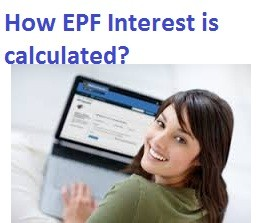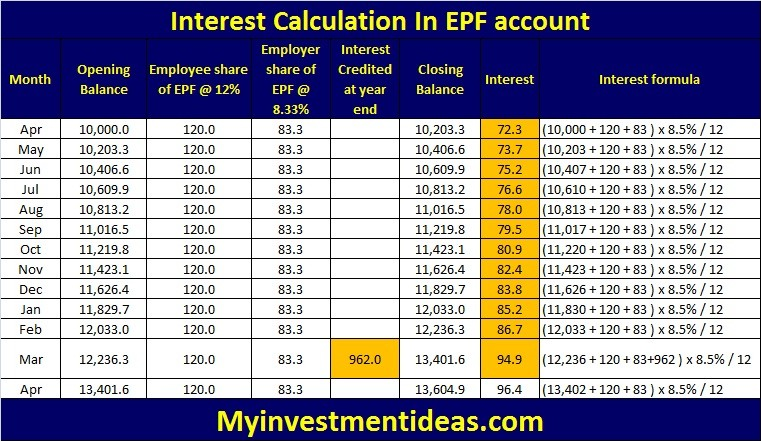# How Employees Provident Fund(EPF) Interest is calculated?How Employees Provident Fund(EPF) Interest is calculated?

As an employee, you would get Provident Fund statement every year. However, you might be wondering on how employees provident fund (EPF) interest is calculated in that statement. What are the components in Provident Fund? Which components in your EPF account affect the interest computation? As an employee, can you try and increase EPF Interest in a financial year? I would provide some insights on EPF interest calculation in this article.

What is Employees Provident Fund (EPF)?

Employee Provident Fund (EPF) is most beneficial and popular retirement saving scheme for salaried individuals. All salaried employees need to contribute 12% of their salary (Basic + DA) to the EPF account every month. While an equal amount of share from an employer would also be contributed, it would get split into 8.33% towards the EPF account and balance 3.67% would be transferred to Employee Pension Scheme (EPS). I would explain more about Employee Pension Scheme (3.67%) contribution of employer through an article in coming weeks.

Also Read: How to check EPF Balance through Mobile?

How Employees Provident Fund (EPF) Interest is calculated?

• Now, let us come to the main point. Interest on EPF is calculated after considering few points.
• Interest on EPF is calculated based on month end balance.
• Month end balance would be equal to opening balance of the month, employee contribution of 12% of salary and 8.33% of employer contribution for the month.
• Interest would be calculated every month, however, credited only at the end of the financial year on 31st March.

EPF Interest Calculation explained with an example.

Related Posts
Deposit Insurance (DICGC) – How far your fixed deposits are safe?

• The balance as of 31st March is Rs 10,000
• Employee basic salary is Rs 1,000 (Basic + DA) per month. So, employee contribution of 12% of Rs 1,000 = Rs 120. Employer contribution of 8.33% of Rs 1,000 = Rs 83.30.
• Assume an EPF interest rate is 8.5% per annum. The monthly interest rate would be 8.5% / 12
• Apr EPF interest = Opening balance + Employee contribution + Employer contribution x Interest rate per month
• Apr EPF interest = (10,000 + 120 + 83.30) x 8.5% / 12 = Rs 72.3
• Now let us come to May. The closing balance of Apr is Rs 10,203.3 (Interest would not be added as it is credited only on 31st March).
• May EPF interest = (10,203.3 + 120 + 83.30) x 8.5% / 12 = Rs 73.7.
• See below table to understand how it would increase month on month. If you observe, interest for the year would be credited only at the year end on 31st March. So interest from Apr to Mar comes to Rs 962 in this case and is credited in Mar month. So Apr of next financial year, the balance in your account would be including interest.As an employee, can you maximise EPF Interest in a financial year?

If you observe above computation, it is clear that month end balance is taken as basis for interest computation. All employers would deposit EPF amount during the month and file statutory filings. The only way your EPF interest would increase is by way of getting salary increments during the year . Otherwise, like in PPF where we can deposit amount before 4th of the month to enjoy interest for that month, in EPF we do not have any other choices to increase the interest.  Alternatively you can contribute to Volutary Provident Fund from employees point of view and get similar interest rate. You can check with your employer whether such facility is available with them.

Suresh
How Employee Provident Fund(EPF) Interest is calculated

• Neeraj Kumar

Sir,

out of 12% employer’s contribution, to the best of my understanding, 8.33% goes to EPS and 3.67% to EPF. Forgive me if I am wrong but I request you to check this once.

Regards

• Vijayan

EPFO is not adding last year’s interest in the current year for the purpose of calculation of interest.
For example, suppose my EPF Balance (Employee share) as on 1.4.17 was 7,00,000 and interest earned in 2016-17 (credited on 31.03.17) was 60,200/- making the total balance as on 1.4.17 to Rs. 760200. The interest @ 8.55 for 2017-18 on this balance should be Rs. 64997 (leave aside the contributions during the year 2017-18). BUT EPF PF STATEMENT SHOWS ONLY RS. 60,675 AS INTEREST WHICH includes 1800 per month contribution for 11 months).

HOW IS EPF INTEREST CALCULATED THEN?